CBSE Class 12 Sample Paper for 2021 Boards

Class 12
Solutions of Sample Papers and Past Year Papers - for Class 12 Boards

## An architect designs a building for a multi-national company. The floor consists of a rectangular region with semicircular ends having a perimeter of 200m as shown below: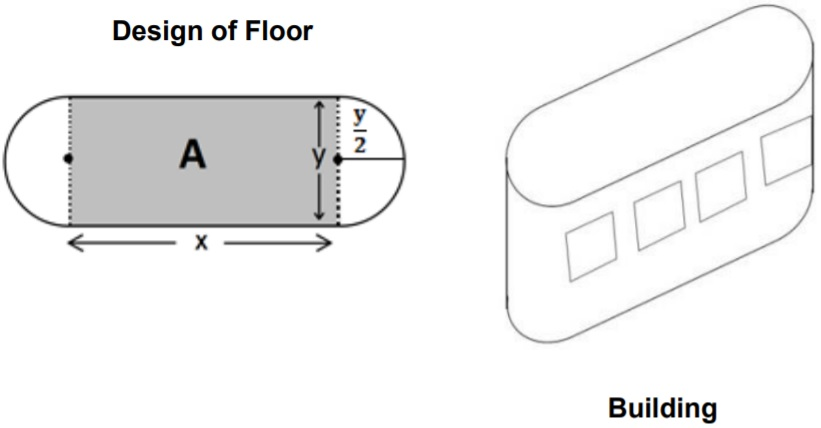## Based on the above information answer the following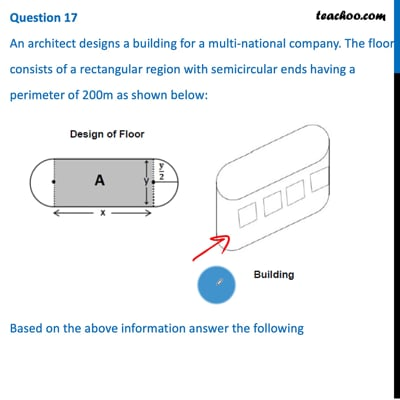This video is only available for Teachoo black users

## d) x + y = 100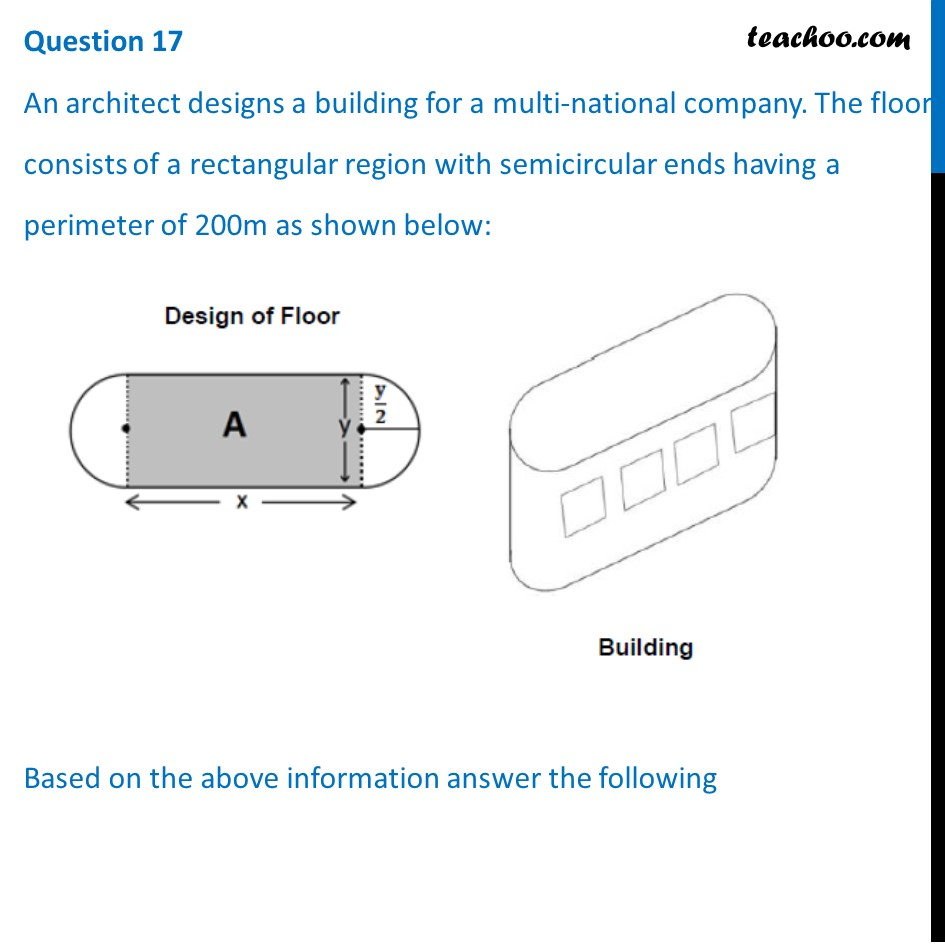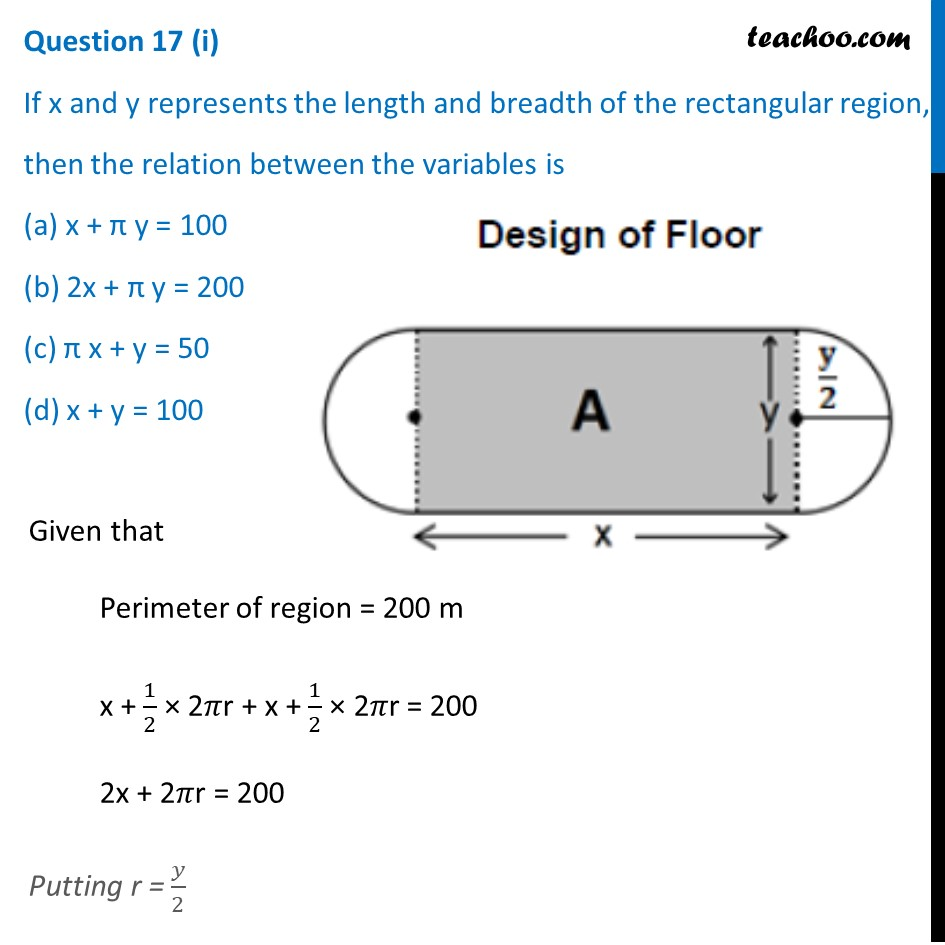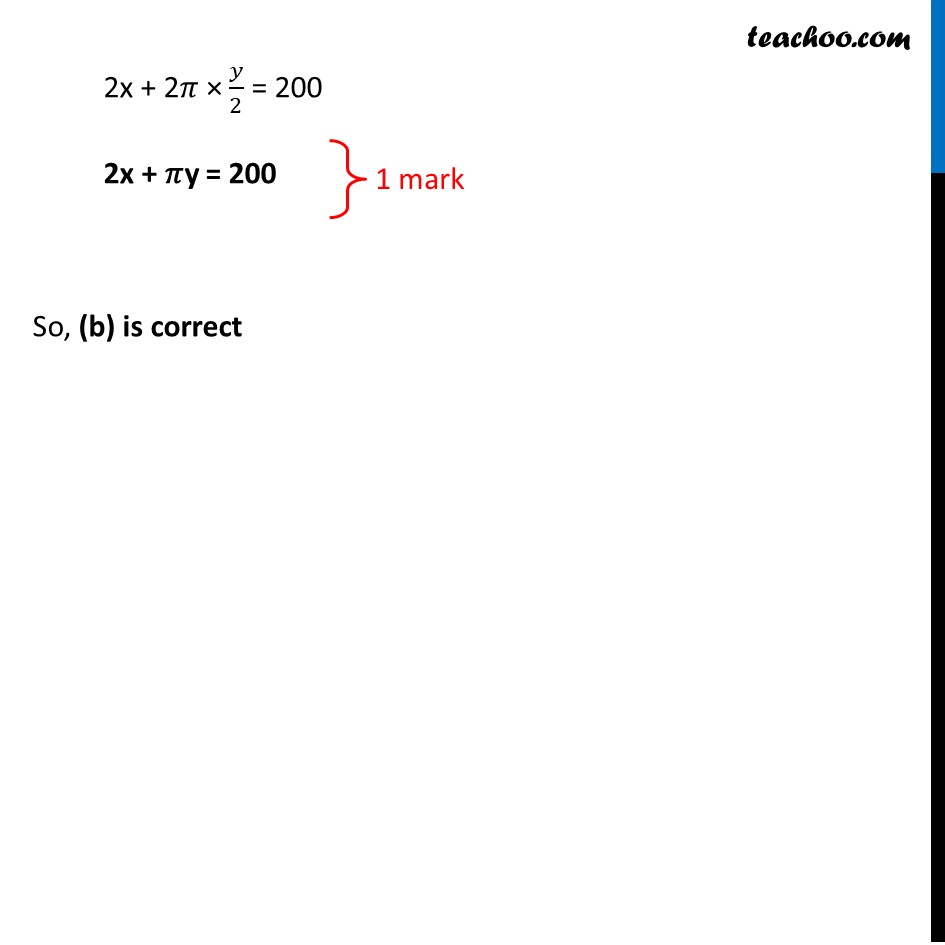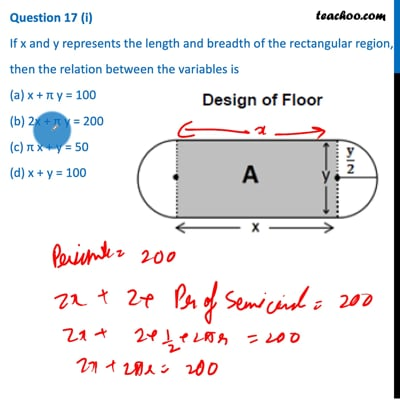This video is only available for Teachoo black users

## d) πy 2 + 2/π (100x – x 2 )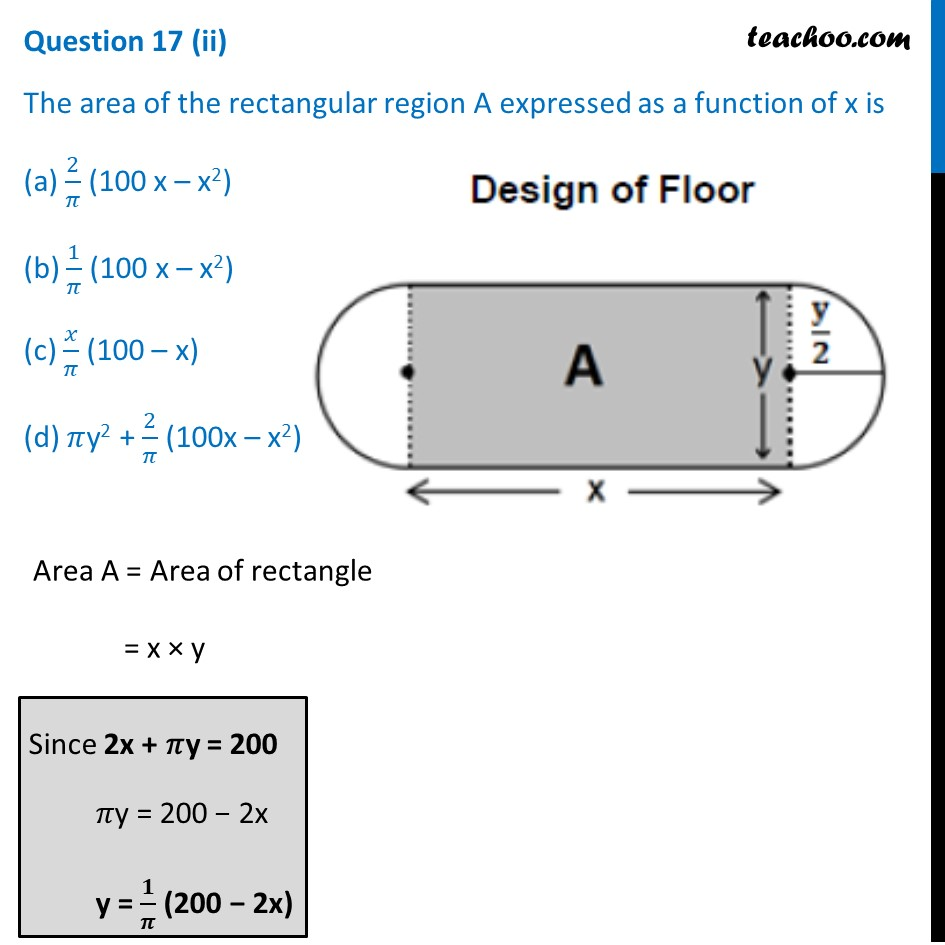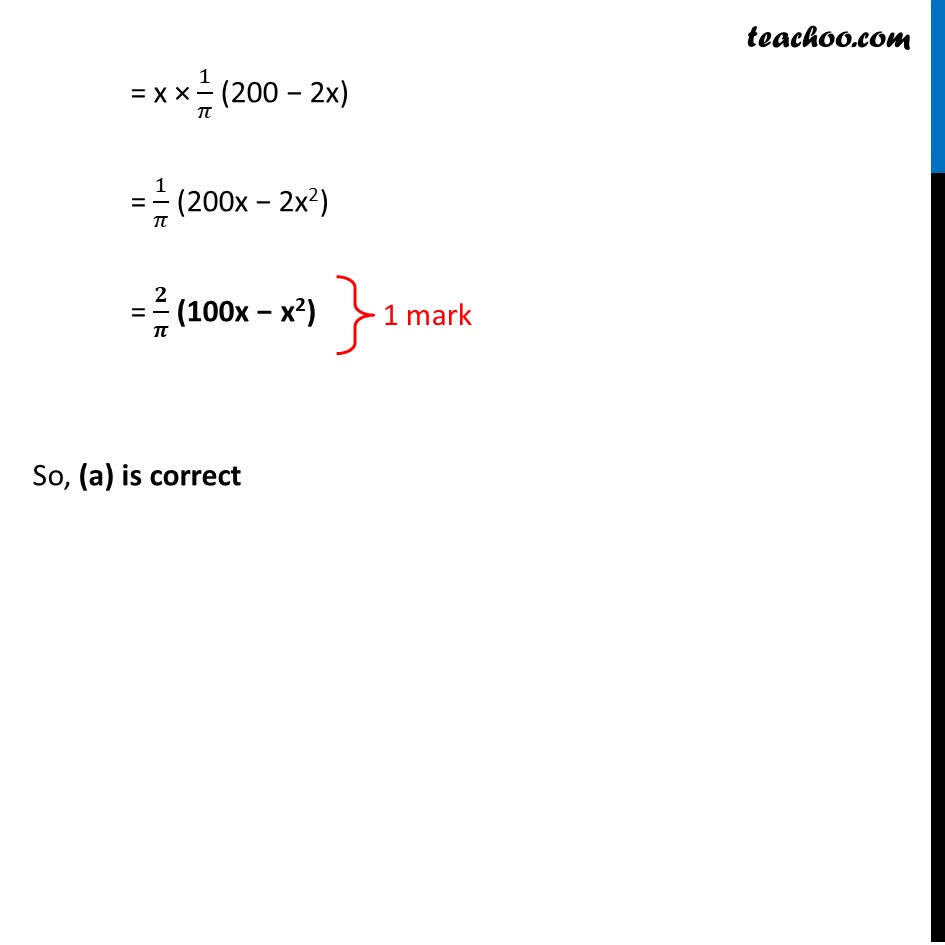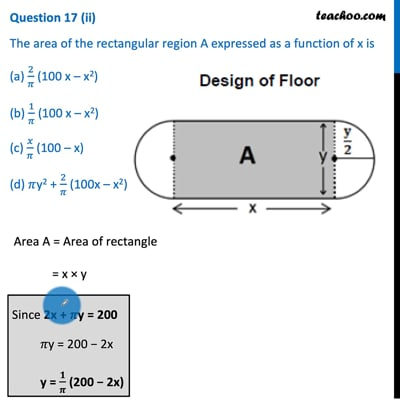This video is only available for Teachoo black users

## d)  1000/π m 2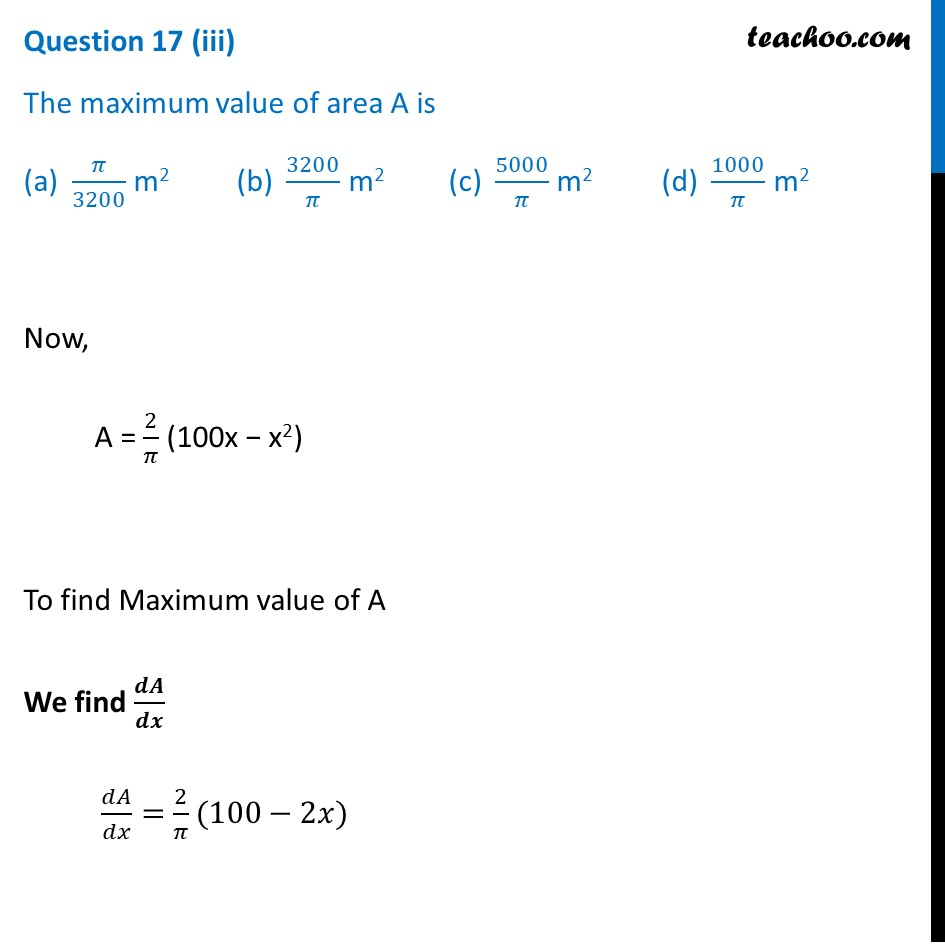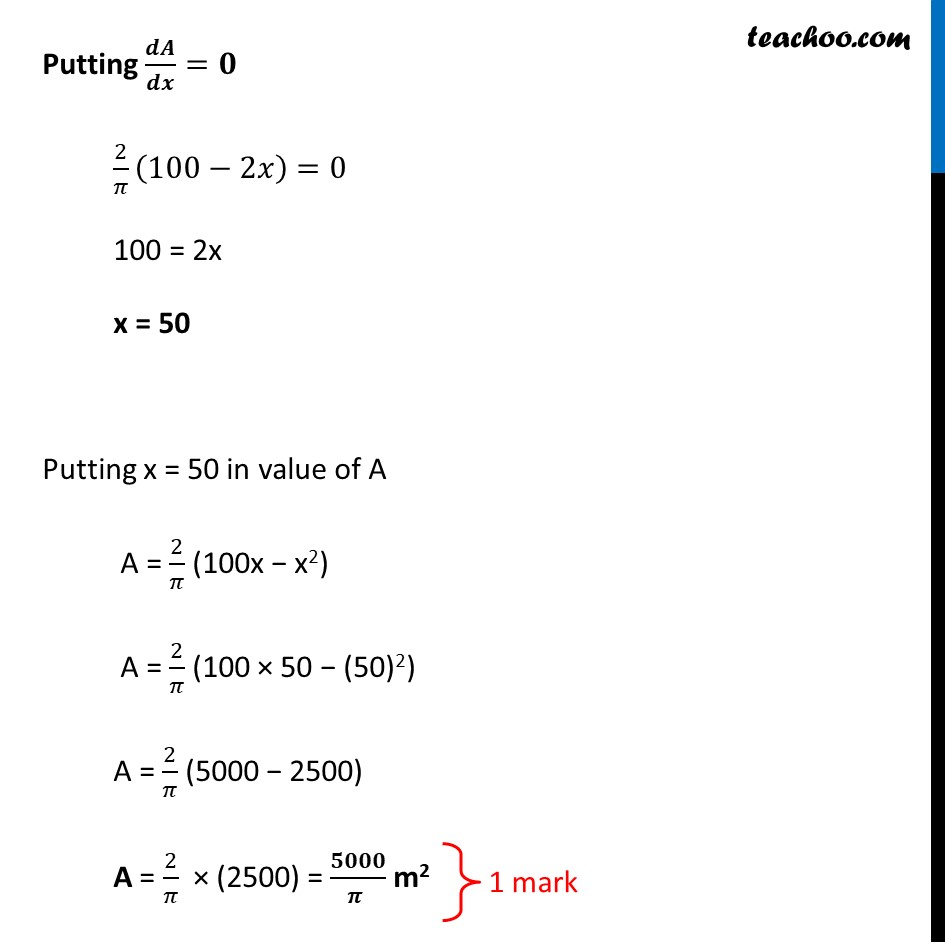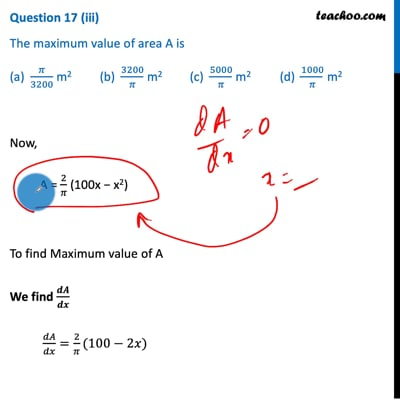This video is only available for Teachoo black users

## (d) 80 m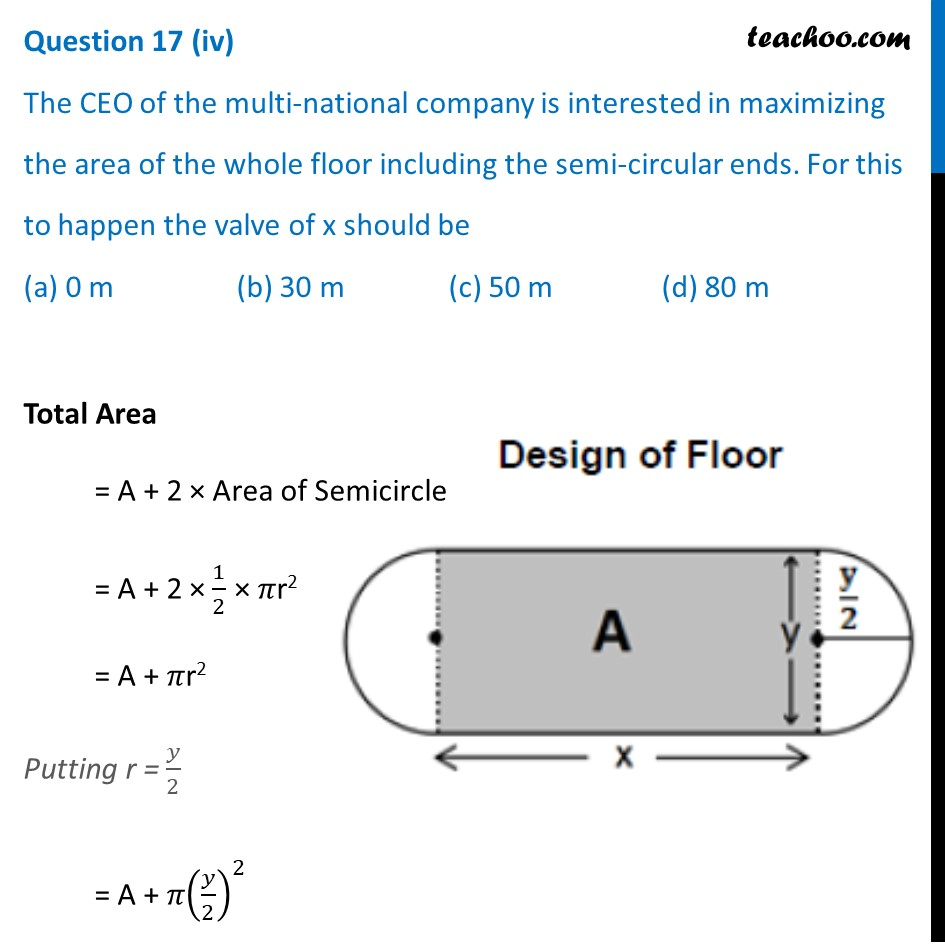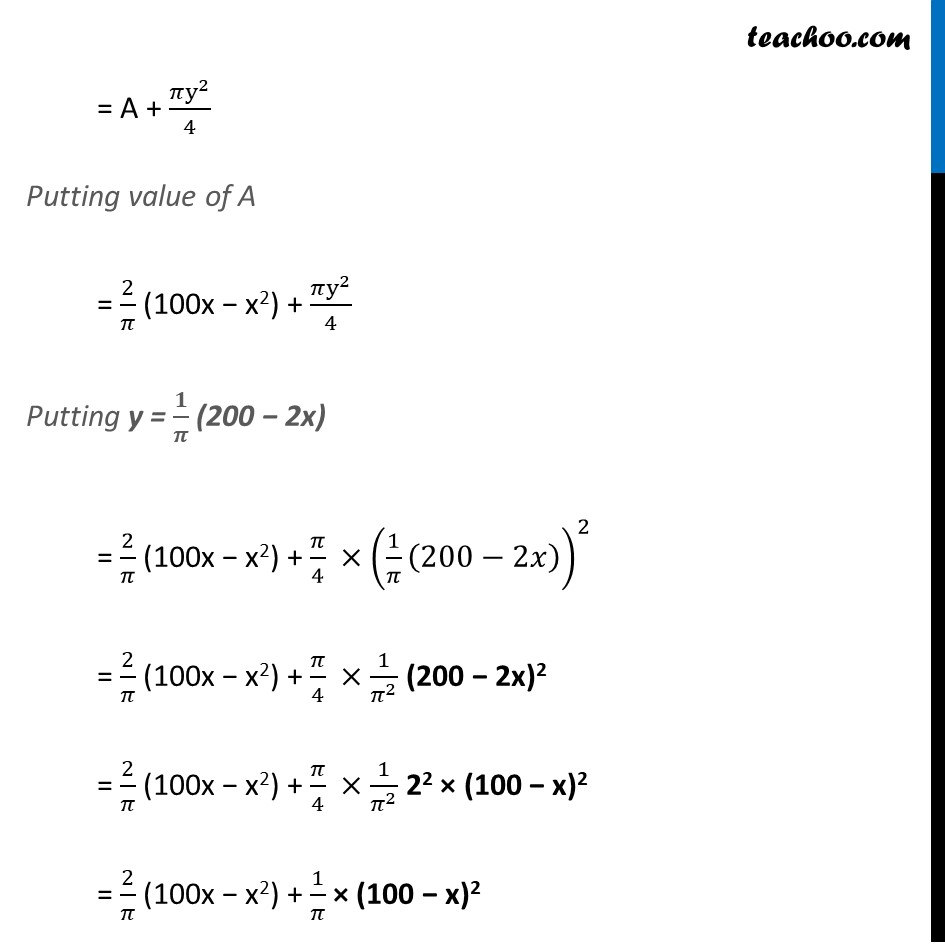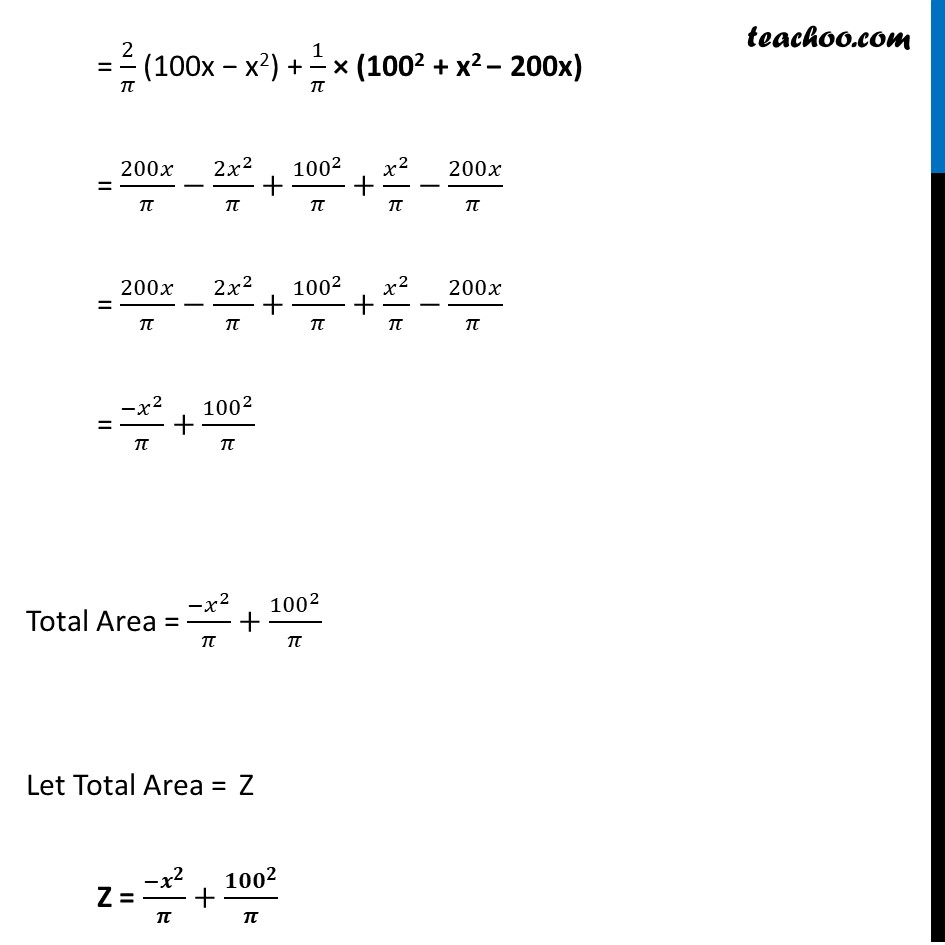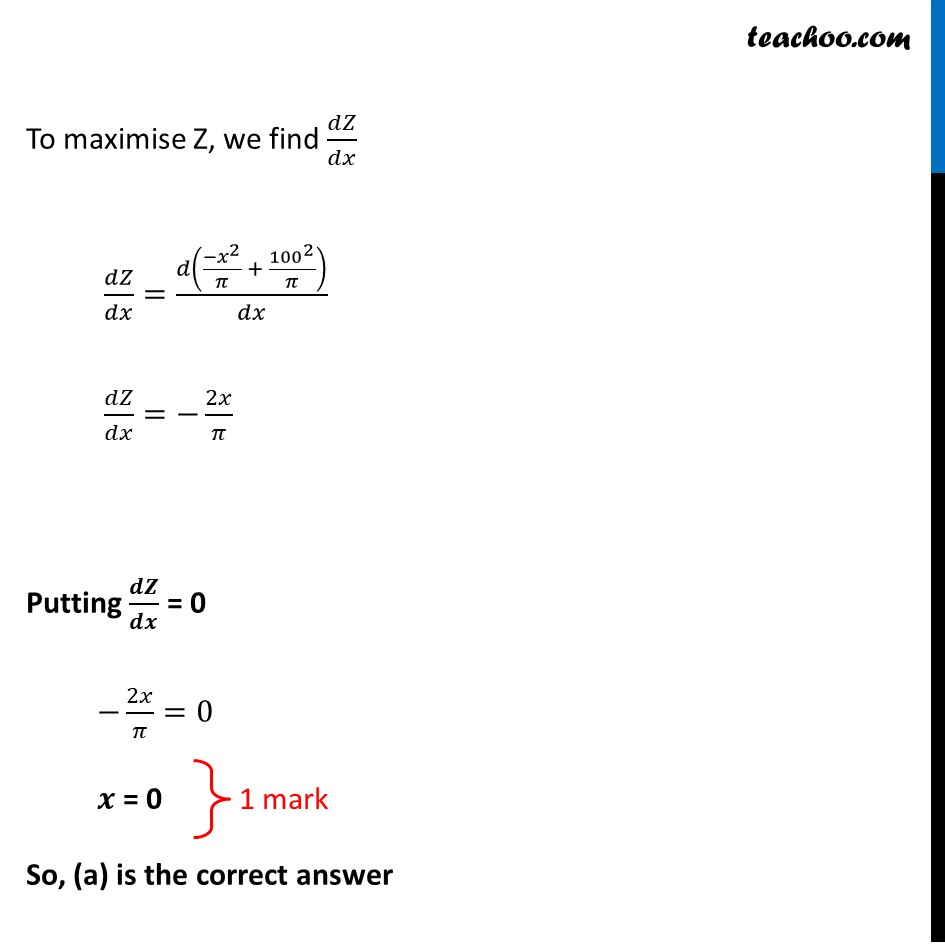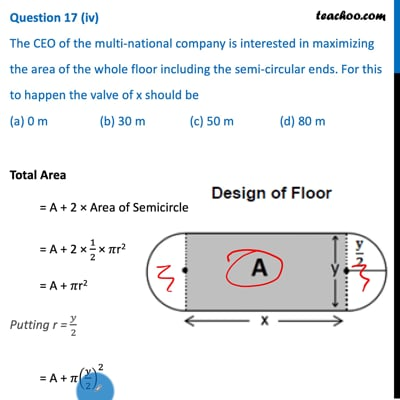This video is only available for Teachoo black users

## (d) No change Both areas are equal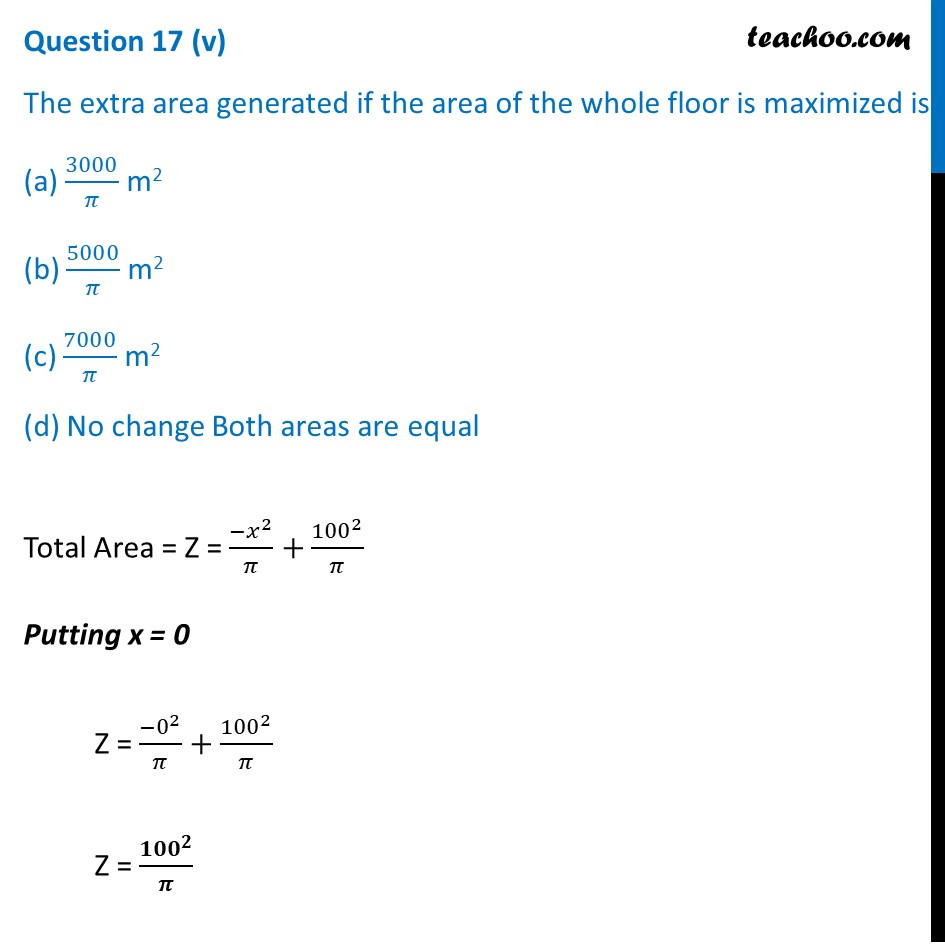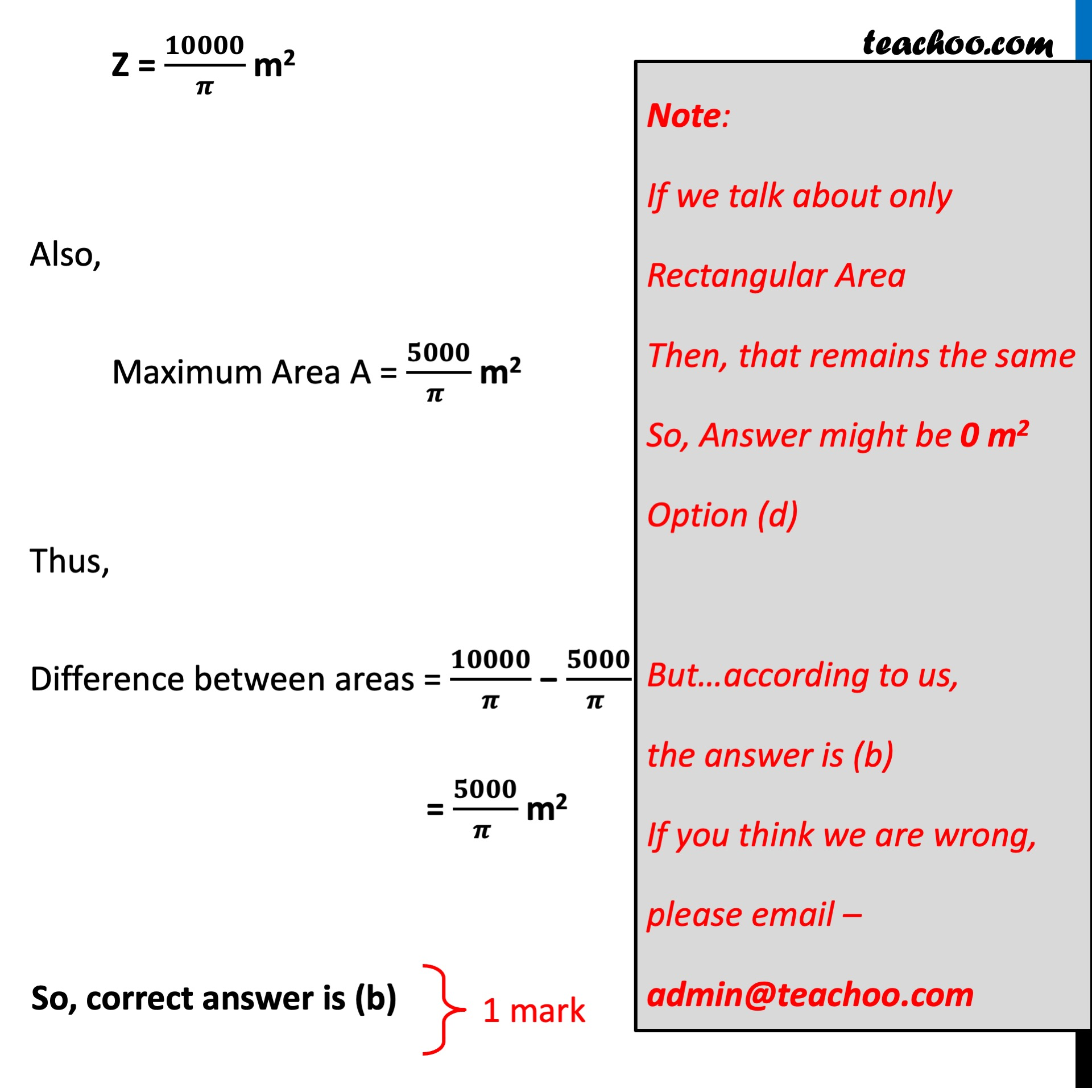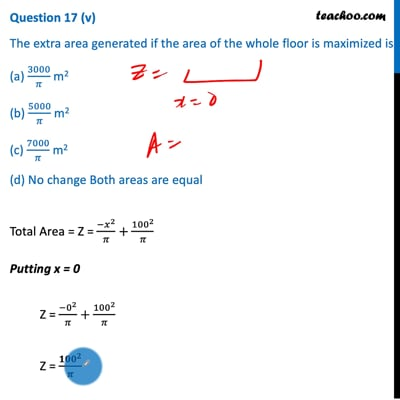This video is only available for Teachoo black users

Introducing your new favourite teacher - Teachoo Black, at only ₹83 per month

### Transcript

Question 17 An architect designs a building for a multi-national company. The floor consists of a rectangular region with semicircular ends having a perimeter of 200m as shown below: Based on the above information answer the following Question 17 (i) If x and y represents the length and breadth of the rectangular region, then the relation between the variables is (a) x + π y = 100 (b) 2x + π y = 200 (c) π x + y = 50 (d) x + y = 100 Given that Perimeter of region = 200 m x + 1/2 × 2𝜋r + x + 1/2 × 2𝜋r = 200 2x + 2𝜋r = 200 Putting r = 𝑦/2 2x + 2𝜋 × 𝑦/2 = 200 2x + 𝜋y = 200 So, (b) is correct Question 17 (ii) The area of the rectangular region A expressed as a function of x is (a) 2/𝜋 (100 x – x2) (b) 1/𝜋 (100 x – x2) (c) 𝑥/𝜋 (100 – x) (d) 𝜋y2 + 2/𝜋 (100x – x2) Area A = Area of rectangle = x × y Since 2x + 𝜋y = 200 𝜋y = 200 − 2x y = 𝟏/𝝅 (200 − 2x) = x × 1/𝜋 (200 − 2x) = 1/𝜋 (200x − 2x2) = 𝟐/𝝅 (100x − x2) So, (a) is correct Question 17 (iii) The maximum value of area A is (a) 𝜋/3200 m2 (b) 3200/𝜋 m2 (c) 5000/𝜋 m2 (d) 1000/𝜋 m2 Now, A = 2/𝜋 (100x − x2) To find Maximum value of A We find 𝒅𝑨/𝒅𝒙 𝑑𝐴/𝑑𝑥=2/𝜋(100−2𝑥) Putting 𝒅𝑨/𝒅𝒙=𝟎 2/𝜋 (100−2𝑥)=0 100 = 2x x = 50 Putting x = 50 in value of A A = 2/𝜋 (100x − x2) A = 2/𝜋 (100 × 50 − (50)2) A = 2/𝜋 (5000 − 2500) A = 2/𝜋 × (2500) = 𝟓𝟎𝟎𝟎/(𝝅 ) m2 Question 17 (iv) The CEO of the multi-national company is interested in maximizing the area of the whole floor including the semi-circular ends. For this to happen the valve of x should be (a) 0 m (b) 30 m (c) 50 m (d) 80 m Total Area = A + 2 × Area of Semicircle = A + 2 × 1/2 × 𝜋r2 = A + 𝜋r2 Putting r = 𝑦/2 = A + 𝜋(𝑦/2)^2 = A + (𝜋y^2)/4 Putting value of A = 2/𝜋 (100x − x2) + (𝜋y^2)/4 Putting y = 𝟏/𝝅 (200 − 2x) = 2/𝜋 (100x − x2) + 𝜋/4 ×(1/𝜋 (200−2𝑥))^2 = 2/𝜋 (100x − x2) + 𝜋/4 ×1/𝜋^2 (200 − 2x)2 = 2/𝜋 (100x − x2) + 𝜋/4 ×1/𝜋^2 22 × (100 − x)2 = 2/𝜋 (100x − x2) + 1/𝜋 × (100 − x)2 = 2/𝜋 (100x − x2) + 1/𝜋 × (1002 + x2 − 200x) = 200𝑥/𝜋−(2𝑥^2)/𝜋+100^2/𝜋+𝑥^2/𝜋−200𝑥/(𝜋 ) = 200𝑥/𝜋−(2𝑥^2)/𝜋+100^2/𝜋+𝑥^2/𝜋−200𝑥/(𝜋 ) = (−𝑥^2)/𝜋+100^2/𝜋 Total Area = (−𝑥^2)/𝜋+100^2/𝜋 Let Total Area = Z Z = (−𝒙^𝟐)/𝝅+〖𝟏𝟎𝟎〗^𝟐/𝝅 To maximise Z, we find 𝑑𝑍/𝑑𝑥 𝑑𝑍/𝑑𝑥=𝑑((−𝑥^2)/𝜋 + 100^2/𝜋)/𝑑𝑥 𝑑𝑍/𝑑𝑥=−2𝑥/𝜋 Putting 𝒅𝒁/𝒅𝒙 = 0 −2𝑥/𝜋=0 𝒙 = 0 So, (a) is the correct answer Question 17 (v) The extra area generated if the area of the whole floor is maximized is (a) 3000/𝜋 m2 (b) 5000/𝜋 m2 (c) 7000/𝜋 m2 (d) No change Both areas are equal Total Area = Z = (−𝑥^2)/𝜋+100^2/𝜋 Putting x = 0 Z = (−0^2)/𝜋+100^2/𝜋 Z = 〖𝟏𝟎𝟎〗^𝟐/𝝅 Z = 𝟏𝟎𝟎𝟎𝟎/𝝅 m2 Also, Maximum Area A = 𝟓𝟎𝟎𝟎/(𝝅 ) m2 Thus, Difference between areas = 𝟏𝟎𝟎𝟎𝟎/𝝅 − 𝟓𝟎𝟎𝟎/(𝝅 ) = 𝟓𝟎𝟎𝟎/(𝝅 ) m2 So, correct answer is (b) Note: This answer doesn’t match the book, if we have done a mistake, please email – admin@teachoo.com